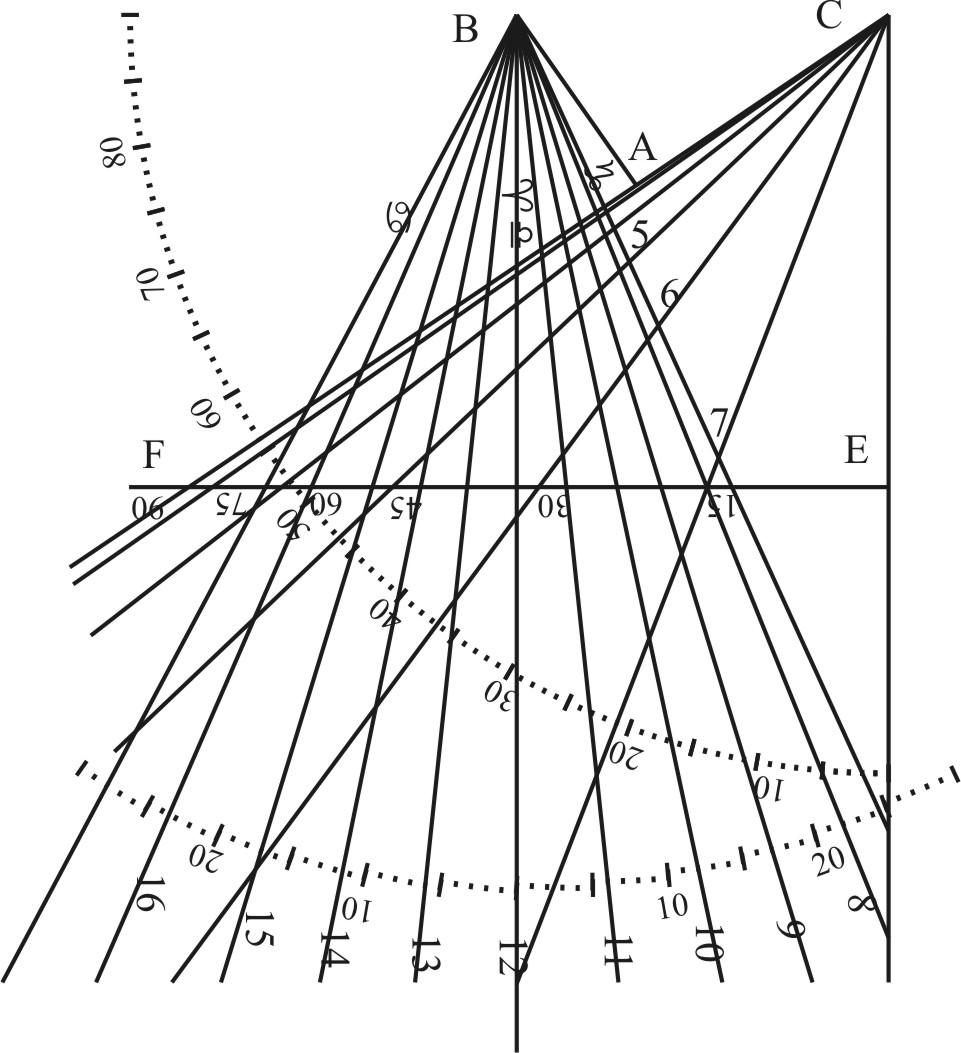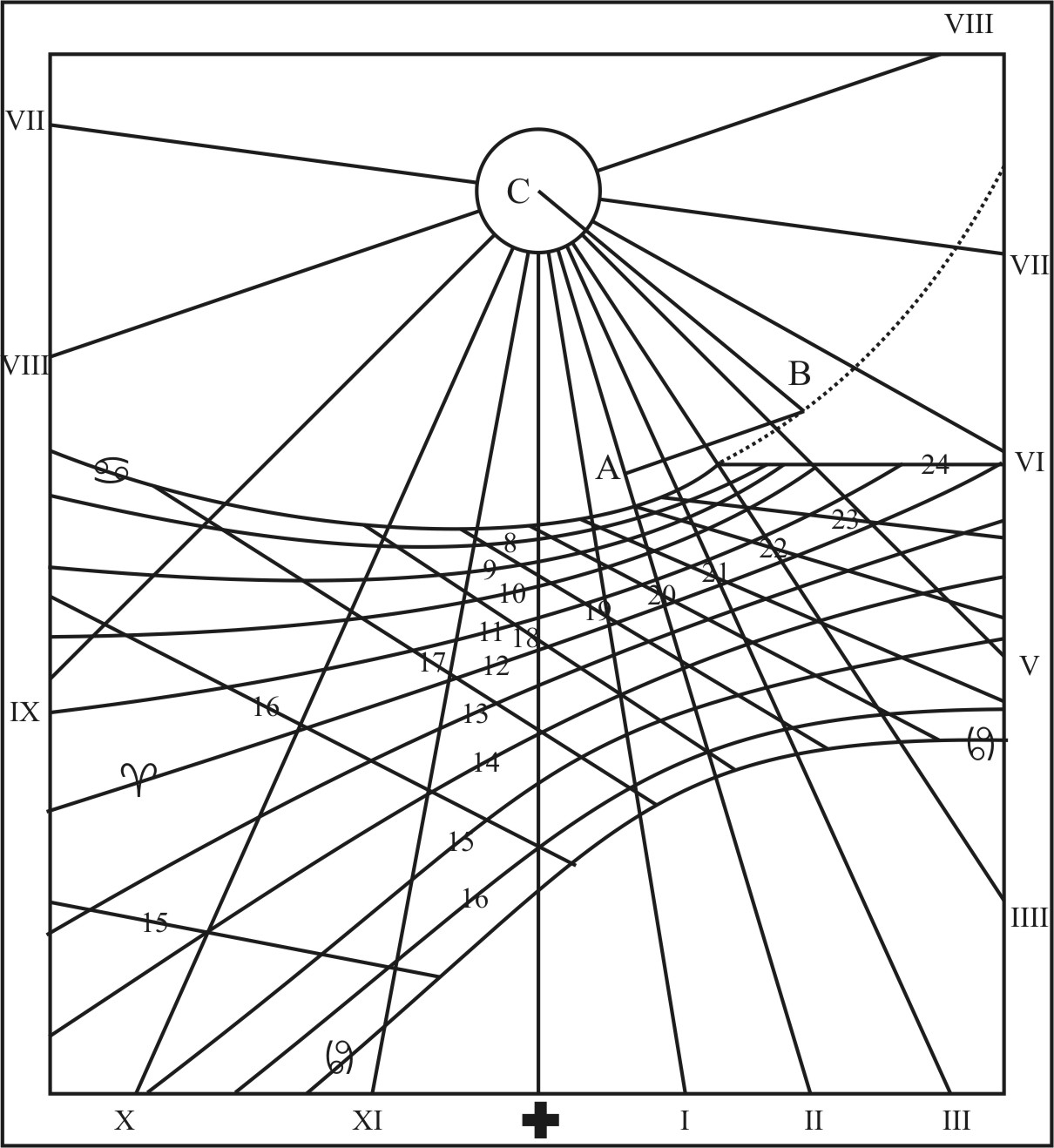CHAP. XV.To describe the Parallels of the length of the day in any of the former Planes.

The length of the Day will always be 12 Hours, when the Sun cometh to be in the Equator, and this holdeth in all Latitudes: but at other times of the Year the same place of the Sun will not give the same length of the Day in other Latitudes; wherefore the Latitude being known, we are first,

To find the Declination of the Sun agreeing to the length of the Day.

Consider the difference between the length of an Equinoctial day, and the day proposed, and turn the time into Degrees and Minutes.

• As the Sine of 90 gr. is to the Sine of half the difference:
• So the Co-tangent of the latitude, to the Tangent of the Declination.As if the length of the Day proposed were 15 Hours, the difference between this and an Equinoctial Day (whose length is always 12 Hours) would be three Hours (which make 45 gr.) and the half difference is 22 gr. 30 m. Wherefore extend the Compasses from the Sine of 90 gr. unto the Tangent of 38 gr. 30 m. the Complement of the latitude, the same extent will reach from the Sine of 22 gr. 30 m. unto the Tangent of 16 gr. 55 m. for the Declination of the Sun at such time as the length of the Day is either 9 or 15 Hours; and from the Sine of 30 gr. unto the Tangent of 21 gr. 40 m. for the Declination belonging to 8 or 16 Hours; and from the Sine of 15 gr. unto the Tangent of 11 gr. 38 m. for the Declination belonging to 10 or 14 Hours; and from the Sine of 7 gr. 30 m. unto the Tangent of 5 gr. 56 m. for the Declination of the Sun when the length of the day is either 11 or 13 Hours.If then you inscribe the Chords of these Arks into the former Figure BDT, the Lines drawn from B through the terms of these Arks shall be the Lines belonging to the Diurnal Arks, and the several Distances between them and the Point C, give the like Distances between the Center and the Parallels of the length of the day upon the Hour-lines in your Plane.

Or comparing these Angles of declination with the Angles at the Equator, you may have the Angels at the Parallel, and then find the Distances between the Center and the Parallel, which being pricked down upon the several Hour-lines, shall give you the Points of Intersection, by which you may draw the parallel of the length of the Day, whereof you have another Example in the Diagram belonging to an Horizontal Plane in Chap. 4. And by the same reason you may draw the Parallels of those Circles to which the Sun is Vertical, the Parallels of the principal Feast, or what else depends on the Declination of the Sun.

Zurück zum Inhaltsverzeichnis Nächstes Kapitel© Rainer Stumpe URL: http://www.rainerstumpe.de